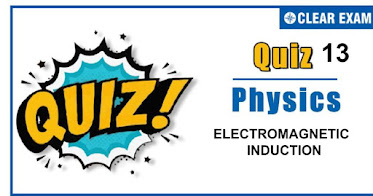## [LATEST]\$type=sticky\$show=home\$rm=0\$va=0\$count=4\$va=0

As per analysis for previous years, it has been observed that students preparing for NEET find Physics out of all the sections to be complex to handle and the majority of them are not able to comprehend the reason behind it. This problem arises especially because these aspirants appearing for the examination are more inclined to have a keen interest in Biology due to their medical background. Furthermore, sections such as Physics are dominantly based on theories, laws, numerical in comparison to a section of Biology which is more of fact-based, life sciences, and includes substantial explanations. By using the table given below, you easily and directly access to the topics and respective links of MCQs. Moreover, to make learning smooth and efficient, all the questions come with their supportive solutions to make utilization of time even more productive. Students will be covered for all their studies as the topics are available from basics to even the most advanced. .

Q1. In a transformer the primary has 500 turnsand secondary has 50 turns. 100 voltsis applied to the primary coil, the voltage developed in the secondary will be
•  1 V
•  10 V
•  1000 V
•  10000 V
Solution
It is a fact.

Q2. Armature current in dc motor will be maximum when
•  Motor has acquired maximum speed
•  Motor has acquired intermediate speed
•  Motor has just started moving
•  Motor is switched off
Solution
Motor e.m.f. equation Eb=V-IaRa At starting Eb=0, so Ia will be maximum

Q3. A short-circulated coil is placed in a time-varying magnetic field. Electrical power is dissipated due to the current induced in the coil. If the number of turns were to be quadrupled and the wire radius halved, the electrical power dissipated would be
•  Halved
•  The same
•  Doubled

Q4. A capacitor is fully charged with a battery. Then the battery is removed and a coil is connected with the capacitor in parallel, current varies as
•  Increases monotonically
•  Decreases monotonically
•  Zero
•  Oscillates indefinitely
Solution
It is a fact.

Q5. In a dc motor, induced e.m.f. will be maximum
•  When motor takes maximum speed
•  When motor starts rotating
•  When speed of motor increases
•  When motor is switched off
Solution
Back emf∝ speed of motor

Q6. The graph gives the magnitude B(t) of a uniform magnetic field that exists throughout a conducting loop, perpendicular to the plane of the loop. Rank the five regions of the graph according to the magnitude of the emf induced in the loop, greatest first
•  b>(d=e)<(a=c)
•  b>(d=e)>(a=c)
• b<d<e<c<a
•  b>(a=c)>(d=e)
Solution
Induced emfe=A dB/dt
i.e.,e∝dB/dt(=slope of B-t graph)
In the given graph slope of AB> slope of CD, slope in the 'a' region = slope in the 'c' region = 0, slope in the 'd' region = slope in the 'e'region ≠0. That’s why b>(d=e)>(a=c)

Q7. In an A.C. generator, when the plane of the armature is perpendicular to the magnetic field
•  Both magnetic flux and emf are maximum
•  Both magnetic flux and emf are zero
•  Both magnetic flux and emf are half of their respective maximum values
•  Magnetic flux is maximum and emf is zero
Solution
Magnetic field, ϕB=BA cos⁡θ Where θ is the angle between normal to the plane of the coil and magnetic field Induced emf, ε=BA sin⁡θ Here, θ=0° ∴ Magnetic flux is maximum and induced emf is zero

Q8. Induced potential in a coil is developed by change of magnetic flux from 1 wb to 0.1wb in 0.1 second is
•  1/9 volt
•  0.09 volt
•  1 volt
•  9 volt
Solution
It is a fact.

Q9. For a large industrial city with much load variations the DC generator should be
•  Series
•  Shunt wound
•  Mixed wound
•  Any
Solution
The DC generator must be mixed wound to withstand the load variation.

Q10.  Consider the situation shown in the figure. The wire AB is sliding on the fixed rails with a constant velocity. If the wire AB is replaced by semicircular wire, the magnitude of the induced current will

•  Increase
•  Remain the same
•  Decrease
• Increase or decrease depending on whether the semicircle bulge is towards the resistance or away from it
Solution
Effective length between A and B remains same## Want to know more

Please fill in the details below:

## Latest NEET Articles\$type=three\$c=3\$author=hide\$comment=hide\$rm=hide\$date=hide\$snippet=hide

Name

ltr
item
BEST NEET COACHING CENTER | BEST IIT JEE COACHING INSTITUTE | BEST NEET, IIT JEE COACHING INSTITUTE: Quiz-13 ELECTROMAGNETIC INDUCTION
Quiz-13 ELECTROMAGNETIC INDUCTION
https://1.bp.blogspot.com/-iZftQeu-E3A/X6EtKxyxobI/AAAAAAAACto/U3PWehkOpuI6TFVeKVUwRQ5Gyk_QqkilQCLcBGAsYHQ/w376-h196/Quiz%2BImage%2B20%2B%25286%2529.jpg
https://1.bp.blogspot.com/-iZftQeu-E3A/X6EtKxyxobI/AAAAAAAACto/U3PWehkOpuI6TFVeKVUwRQ5Gyk_QqkilQCLcBGAsYHQ/s72-w376-c-h196/Quiz%2BImage%2B20%2B%25286%2529.jpg
BEST NEET COACHING CENTER | BEST IIT JEE COACHING INSTITUTE | BEST NEET, IIT JEE COACHING INSTITUTE
https://www.cleariitmedical.com/2020/11/quiz-13-electromagnetic-induction.html
https://www.cleariitmedical.com/
https://www.cleariitmedical.com/
https://www.cleariitmedical.com/2020/11/quiz-13-electromagnetic-induction.html
true
7783647550433378923
UTF-8